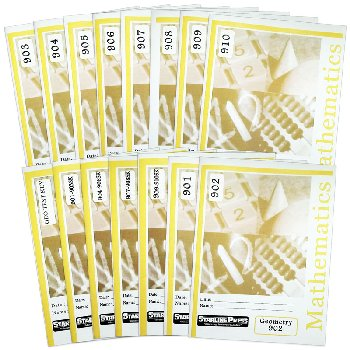# Geometry Set

# BM-051457

Bargain Price: \$126.94
Retail: \$186.72
Save: 32.02% (\$59.78)
1 Bargain Versions available. Non-returnable.
Qty:Item #: BM-051457 9

#### Product Description:

GEOMETRY: Covers Geometry basics: points and lines, line segments, rays, planes, and lines and points in planes. Students study definitions of angles, degrees, and measuring the size of angles. They are taught to classify angles by size and relation and how to bisect an angle. Students study parallelograms, properties of a trapezoid, ration and proportions, and the Pythagorean Theorem. Ninth grade students know, derive, and solve problems involving perimeter, circumference, area, volume, lateral area and surface area of common geometric features. Students know the definitions of basic trigonometric functions defined by the angles of a right triangle. They also know and are able to use elementary relationships between functions.

#### Publisher Description:

Ninth grade students learn points and lines, line segments, rays, planes, and lines and points in planes. They study definitions of angles and degrees and measuring the size of angles. They learn to classify angles by size and relation and how to bisect an angle. Students study parallelograms, properties of a trapezoid, ratio and proportions, and the Pythagorean Theorem. Ninth grade students know derive and solve problems involving the perimeter, circumference, area, volume, lateral area, and surface area of common geometric figures. Students know the definitions of basic trigonometric functions defined by the angles of a right triangle. They also know and are able to use elementary relationships between functions.

Primary Subject
Mathematics
9
Format
Softcover Book Set
Brand Name
Starline Press
Weight
4.7 (lbs.)
Dimensions
11.0" x 8.5" x 2.5"